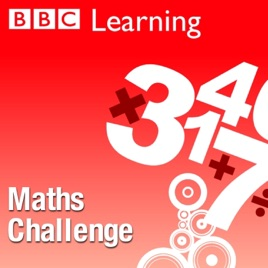19エピソード

Maths quizzes for children aged 9 - 11 emphasising mental maths and problem solving.# Maths Challenge BBC

• 教育

Maths quizzes for children aged 9 - 11 emphasising mental maths and problem solving.

Equivalent fractions

## Equivalent fractions

Ralph van Dijk returns with a quiz with questions about equivalent fractions.

• 14分
Dividing tenths and hundredths

## Dividing tenths and hundredths

Ralph van Dijk returns with a quiz with questions about dividing tenths and hundredths.

• 13分
Multiplying with zeroes

## Multiplying with zeroes

Ralph van Dijk returns with a quiz with questions about multiplying with zeroes.

• 14分
Calculating with large numbers

## Calculating with large numbers

Ralph van Dijk returns with a quiz with questions about calculating with large numbers.

• 14分
Rounding numbers up and down

## Rounding numbers up and down

Ralph van Dijk returns with a quiz with questions about rounding numbers up and down.

• 14分